# Definition

`Parallel plot` or parallel coordinates plot allows to compare the feature of several individual observations (`series`) on a set of numeric variables. Each vertical bar represents a variable and often has its own scale. (The units can even be different). Values are then plotted as series of lines connected across each axis.

The `ìris` dataset provides four features (each represented with a vertical line) for 150 flower samples (each represented with a color line). Samples are grouped in three species. The chart below highlights efficiently that setosa has smaller Petals, but its sepal tends to be wider.

``````# Libraries
library(tidyverse)
library(hrbrthemes)
library(patchwork)
library(GGally)
library(viridis)

# Data set is provided by R natively
data <- iris

# Plot
data %>%
ggparcoord(
columns = 1:4, groupColumn = 5, order = "anyClass",
showPoints = TRUE,
title = "Parallel Coordinate Plot for the Iris Data",
alphaLines = 0.3
) +
scale_color_viridis(discrete=TRUE) +
theme_ipsum()+
theme(
plot.title = element_text(size=10)
)``````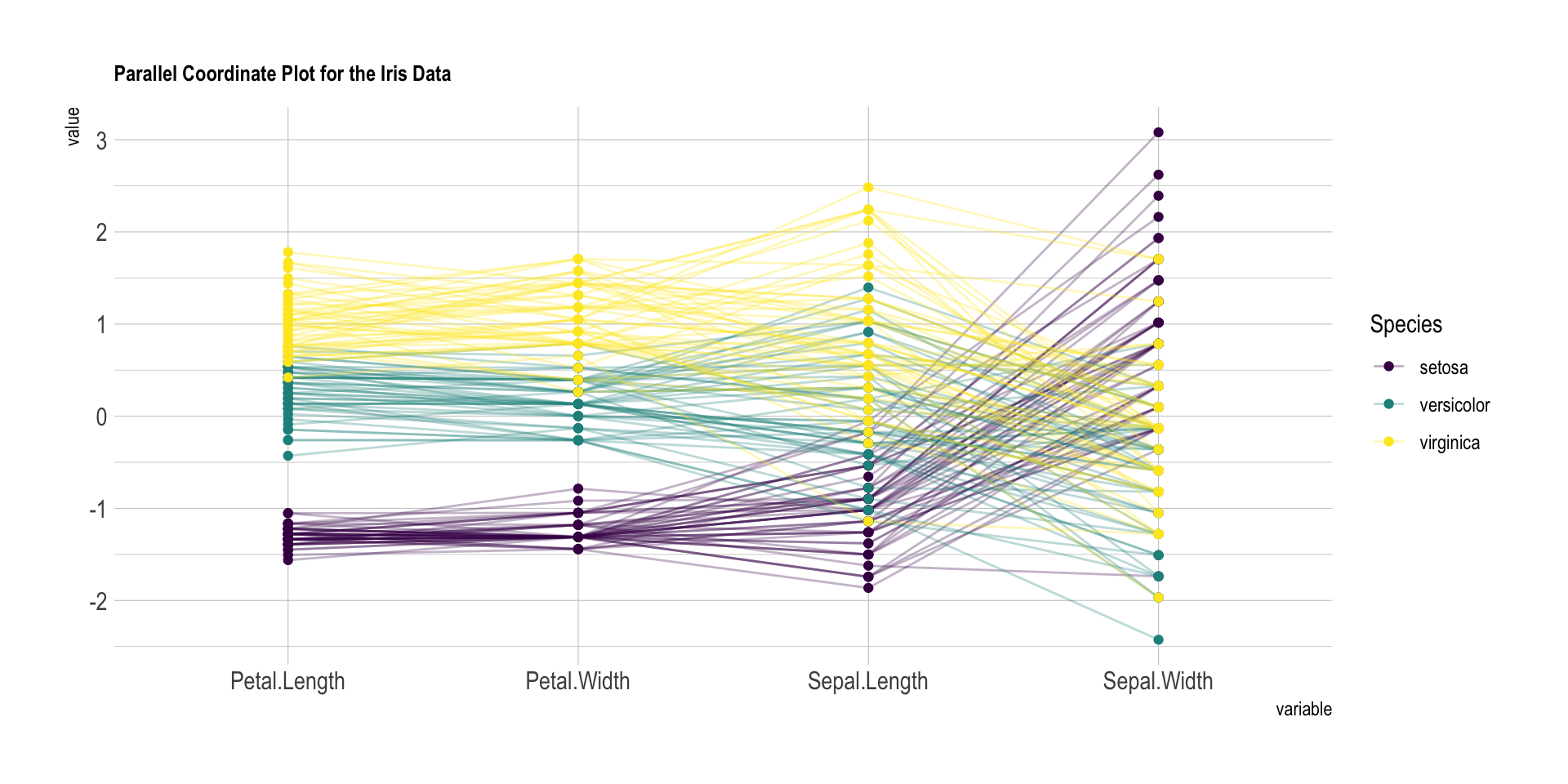Note: Parallel plot is the equivalent of a spider chart, but with cartesian coordinates. Thus, it is often prefered.

# What for

A parallel plot allows to study the features of samples for `several quantitative` variables. Its strength is that the variables can even be completely different: different `ranges` and even different `units`.

In the graphic above flower features were grouped in species, and all variables were normalized and sharing the same unit (cm). Here is another example where diamonds are compared for 4 variables that share different units, like the price in \$ or depth in %. Note the use of scaling to be able to compare them.

``````diamonds %>%
sample_n(10) %>%
ggparcoord(
columns = c(1,5:7),
groupColumn = 2,
#order = "anyClass",
showPoints = TRUE,
title = "Diamonds features",
alphaLines = 0.3
) +
scale_color_viridis(discrete=TRUE) +
theme_ipsum()+
theme(
plot.title = element_text(size=10)
)``````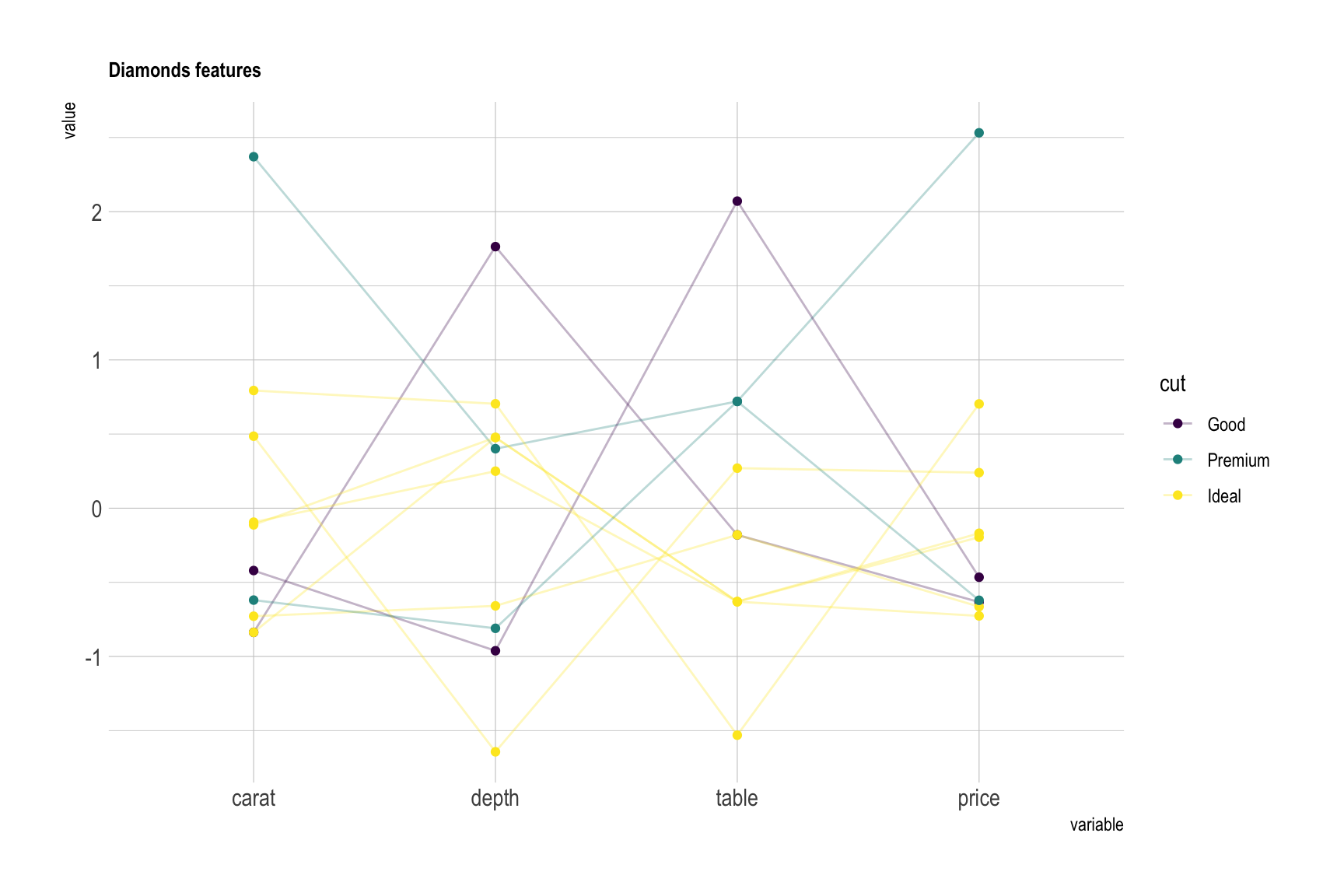# Variation

Here is an overview of the parallel coordinates features you can play with:

• Scaling - scaling transforms the raw data to a new scale that is common with other variables. It is a crucial step to compare variables that do not have the same unit, but can also help otherwise as shown in the example below:
``````# Plot
p1 <- data %>%
ggparcoord(
columns = 1:4, groupColumn = 5, order = "anyClass",
scale="globalminmax",
showPoints = TRUE,
title = "No scaling",
alphaLines = 0.3
) +
scale_color_viridis(discrete=TRUE) +
theme_ipsum()+
theme(
legend.position="none",
plot.title = element_text(size=10)
) +
xlab("")

p2 <- data %>%
ggparcoord(
columns = 1:4, groupColumn = 5, order = "anyClass",
scale="uniminmax",
showPoints = TRUE,
title = "Standardize to Min = 0 and Max = 1",
alphaLines = 0.3
) +
scale_color_viridis(discrete=TRUE) +
theme_ipsum()+
theme(
legend.position="none",
plot.title = element_text(size=10)
) +
xlab("")

p3 <- data %>%
ggparcoord(
columns = 1:4, groupColumn = 5, order = "anyClass",
scale="std",
showPoints = TRUE,
title = "Normalize univariately (substract mean & divide by sd)",
alphaLines = 0.3
) +
scale_color_viridis(discrete=TRUE) +
theme_ipsum()+
theme(
legend.position="none",
plot.title = element_text(size=10)
) +
xlab("")

p4 <- data %>%
ggparcoord(
columns = 1:4, groupColumn = 5, order = "anyClass",
scale="center",
showPoints = TRUE,
title = "Standardize and center variables",
alphaLines = 0.3
) +
scale_color_viridis(discrete=TRUE) +
theme_ipsum()+
theme(
legend.position="none",
plot.title = element_text(size=10)
) +
xlab("")

p1 + p2 + p3 + p4 + plot_layout(ncol = 2)``````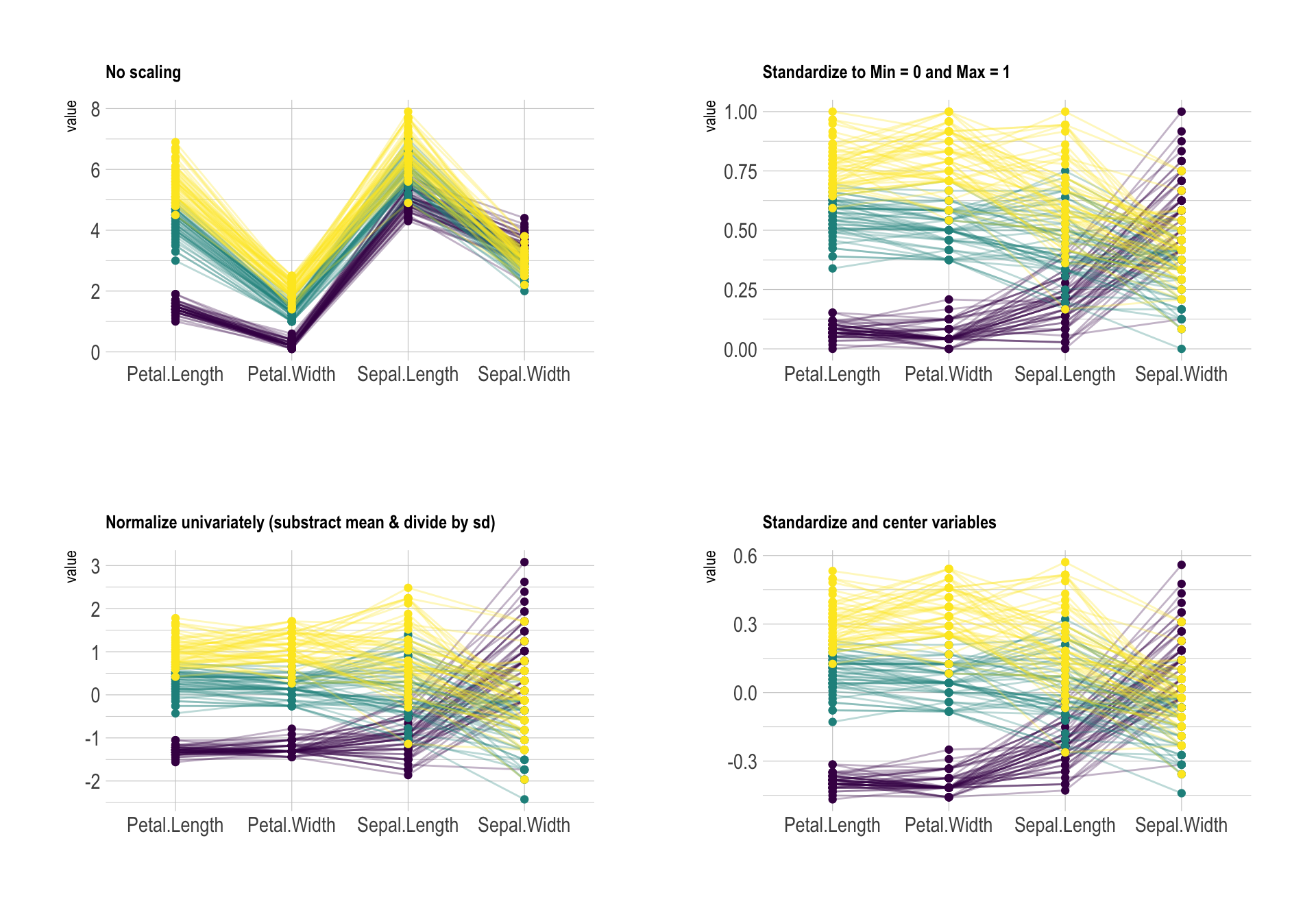• Axis order - optimizing the order of vertical axis can decrease the `clutter` of your parallel plot. Basically, the goal is to minimize the number of cross between series. On the next figure, the left plot is much harder to understand the the right one. Only variable order is different.
``````# Plot
p1 <- data %>%
ggparcoord(
columns = 1:4, groupColumn = 5, order = c(1:4),
showPoints = TRUE,
title = "Original",
alphaLines = 0.3
) +
scale_color_viridis(discrete=TRUE) +
theme_ipsum()+
theme(
legend.position="Default",
plot.title = element_text(size=10)
) +
xlab("")

p2 <- data %>%
ggparcoord(
columns = 1:4, groupColumn = 5, order = "anyClass",
showPoints = TRUE,
title = "Re-ordered",
alphaLines = 0.3
) +
scale_color_viridis(discrete=TRUE) +
theme_ipsum()+
theme(
legend.position="none",
plot.title = element_text(size=10)
) +
xlab("")

p1 + p2 ``````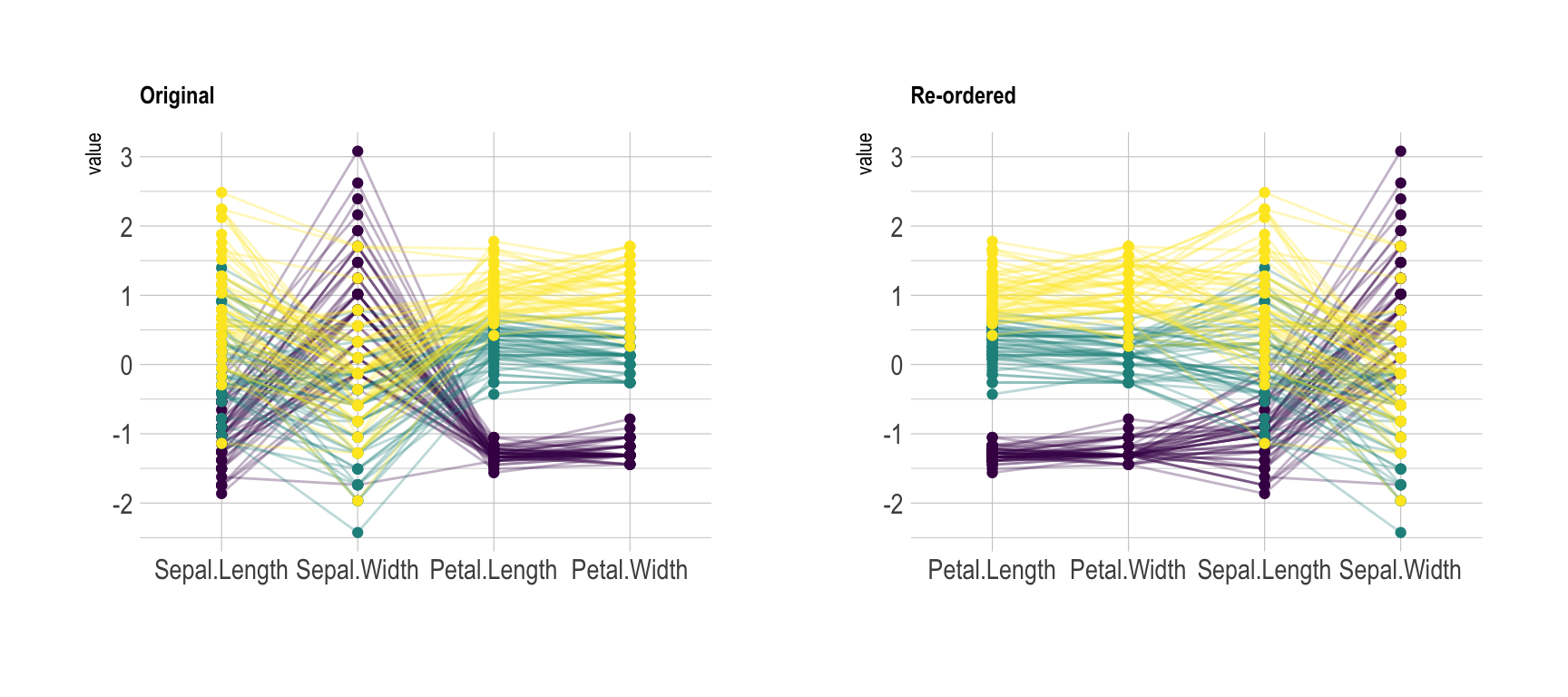• Highlighting - a parallel plot being a line plot, the main caveat is the spaghetti chart where too many lines overlap, making the chart unreadable. Several workaround exist as described in this page. A solution is to highlight a specific sample or a specific group of interest:
``````# Plot
data %>%
ggparcoord(
columns = 1:4, groupColumn = 5, order = "anyClass",
showPoints = TRUE,
title = "Original",
alphaLines = 0.3
) +
scale_color_manual(values=c( "#69b3a2", "grey", "grey") ) +
theme_ipsum()+
theme(
legend.position="Default",
plot.title = element_text(size=10)
) +
xlab("")``````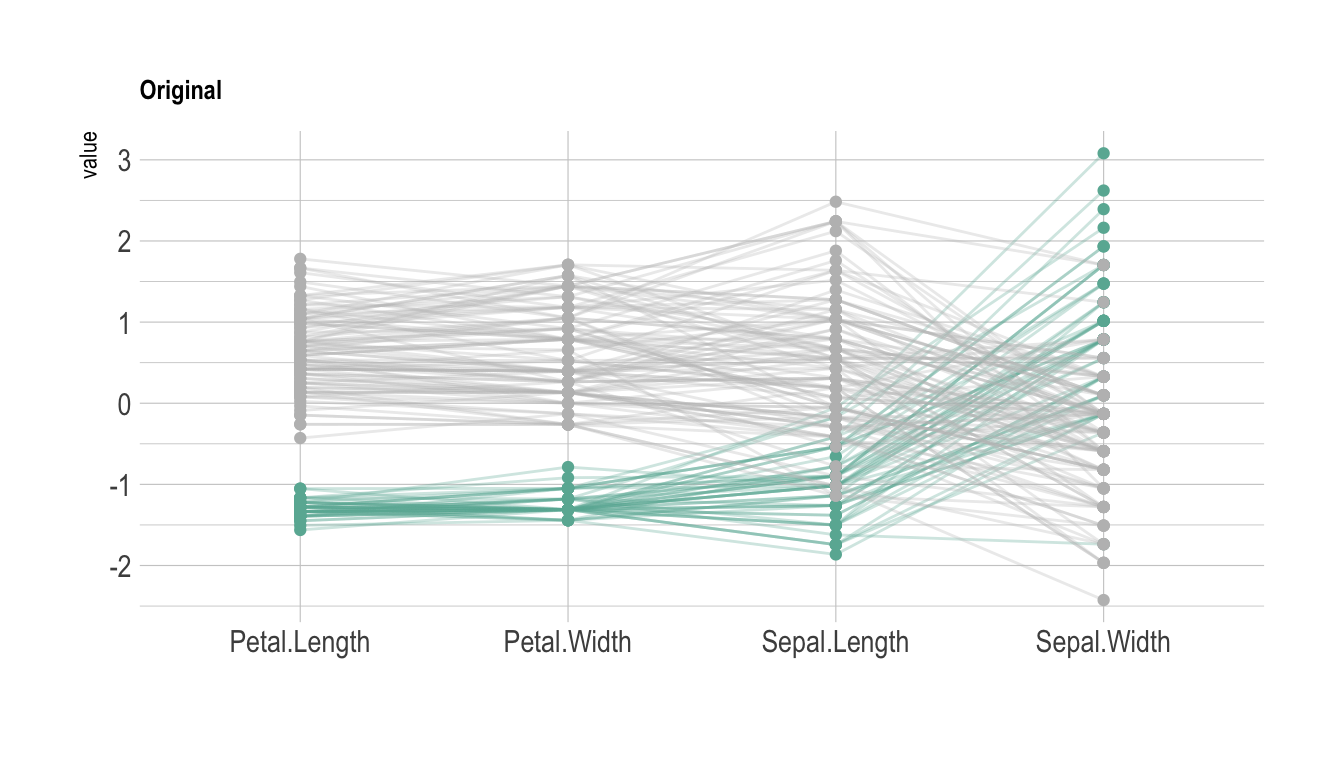# Common mistakes

• Like for line plot, displaying too many samples result in a cluttered and unreadable spaghetti chart.
• Sort the variables on the X axis, it makes sense to avoid crosses in sample lines.
• Try different scalings to find the one fitting your data best.

# Related

The R and Python graph galleries are 2 websites providing hundreds of chart example, always providing the reproducible code. Click the button below to see how to build the chart you need with your favorite programing language.